Courses

# Chemistry- CY 2015 GATE Paper (Practice Test)

## 65 Questions MCQ Test GATE Past Year Papers for Practice (All Branches) | Chemistry- CY 2015 GATE Paper (Practice Test)

Description
This mock test of Chemistry- CY 2015 GATE Paper (Practice Test) for GATE helps you for every GATE entrance exam. This contains 65 Multiple Choice Questions for GATE Chemistry- CY 2015 GATE Paper (Practice Test) (mcq) to study with solutions a complete question bank. The solved questions answers in this Chemistry- CY 2015 GATE Paper (Practice Test) quiz give you a good mix of easy questions and tough questions. GATE students definitely take this Chemistry- CY 2015 GATE Paper (Practice Test) exercise for a better result in the exam. You can find other Chemistry- CY 2015 GATE Paper (Practice Test) extra questions, long questions & short questions for GATE on EduRev as well by searching above.
QUESTION: 1

Solution:
QUESTION: 2

Solution:
QUESTION: 3

### Choose the most similar in meaning to the given word. Educe

Solution:
QUESTION: 4

Operators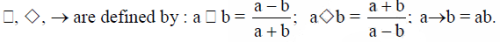Find the value of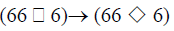Solution: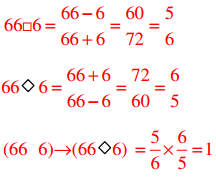QUESTION: 5

if logx(5/7) = -1/3, then the value of x is

Solution:
QUESTION: 6

Q. 6 – Q. 10 carry Two mark each.

Q.

The following question presents a sentence, part of which is underlined. Beneath the sentence you find four ways of pharasing the underlined part. Following the requirements of the standard written english, select the answer that produces the most effective sentence.

Tuberculosis, together with its effect, "ranks one of the leading cause of death" in India

Solution:
QUESTION: 7

Read the following paragraph and choose the correct statement.

climate change has reduced human security and threatened human well being. An ignored reality of human progress is that human securiy largely depends upon environmental security. But on the contrary, human progress seems contradictory to environmental security. To keep up both at the required level is a challange to be addressed by one and all. One of the ways to curb the climate change may be suitable scientific innovations, while the other way may be the Gadhian perspective on small scale progress wih focus on sustainability.

Solution:
*Answer can only contain numeric values
QUESTION: 8

find the missing value.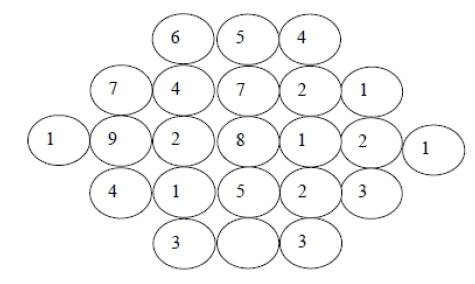Solution:
QUESTION: 9

Acube of side 3 units is formed using a set of smaller cubes of side 1 unit. Find the proportion of the number of faces of the smaller cubes visible to those which are NOT visible.

Solution:
QUESTION: 10

Hummpty Dumpty sits on a wall every day while having lunch. the wall sometimes breaks. A person sitting on the wall falls if the wall breaks.

Which one of the statements below is logically valid and can be inferred from te above sentences.?

Solution:
QUESTION: 11

Q. 1 – Q. 25 carry one mark each

Q.

Which one of the following plots represents an acceptable wavefunction?

Solution:
QUESTION: 12

When the operator, –h2d2/dx2, operates on the function eikx, the result is

Solution:
QUESTION: 13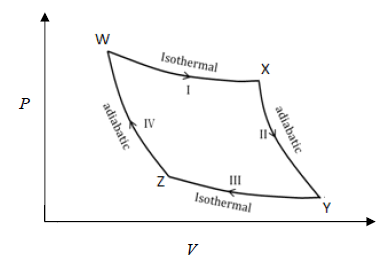From the above Carnot cycle undergone by an ideal gas, identify the processes in which the change in internal energy is NON-ZERO.

Solution:
QUESTION: 14

For an ideal gas with molar mass M, the molar translational entropy at a given temperature is proportional to

Solution:
QUESTION: 15

Which one of the following defines the absolute temperature of a system?

Solution:
QUESTION: 16

Which of theollowing properties are characteristic of an ideal solution?

(i) (ΔmixG)T, P is negative

(ii) (ΔmixS)T, P is positive

(iii) (ΔmixV)T, P is positive

(iv) (ΔmixH)T, P is negative

Solution:
QUESTION: 17

The expression for the equilibrium constant (Keq) for the enzyme catalyzed reaction given below, is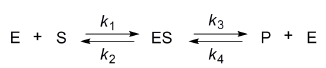Solution:
*Answer can only contain numeric values
QUESTION: 18

Given the E0 values for the following reaction sequence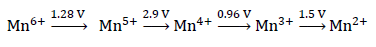the computed value of E0 for Mn6+→Mn2+ (in volts) is _______________

Solution:
QUESTION: 19

The absorption spectrum of [Ti(H2O)6]3+ in solution comprises of a maximum with a shoulder. The reason for the shoulder is

Solution:
QUESTION: 20

The ease of formation of the adduct, NH3·BX3 (where, X = F, Cl, Br) follows the order

Solution:
QUESTION: 21

An efficient catalyst for hydrogenation of alkenes is [Rh(PPh3)3Cl]. However, [Ir(PPh3)3Cl] does not catalyze this reaction, because

Solution:
QUESTION: 22

Among the given pH values, the O2 binding efficiency of hemoglobin is maximum at

Solution:
QUESTION: 23

The intense red color of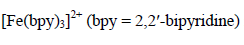is due to

Solution:
QUESTION: 24

The compound with planar geometry is

Solution:
QUESTION: 25

The electrical conductivity of a metal

Solution:
QUESTION: 26

Which one of the following statements is INCORRECT?

Solution:
QUESTION: 27

The absolute configuration of C2 and C3 in the following compound is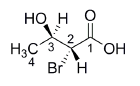Solution:
QUESTION: 28

Among the following compounds, the one that is non-aromatic, is

Solution:
QUESTION: 29

The correct order of reactivity of p-halonitrobenzenes in the following reaction is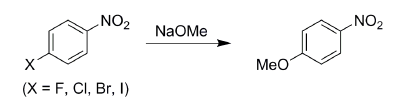Solution:
QUESTION: 30

Tollen’s test is NEGATIVE for

Solution:
QUESTION: 31

The compound given below is a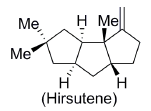Solution:
QUESTION: 32

Amongst the following, the compound that DOES NOT act as a diene in Diels-Alder reaction is

Solution:
QUESTION: 33

The following conversion is an example of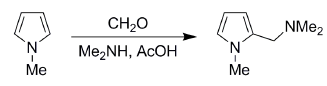Solution:
QUESTION: 34

The mass spectrum of a dihalo compound shows peaks with relative intensities of 1:2:1 corresponding to M, M+2 and M+4 (M is the mass of the molecular ion), respectively. The compound is

Solution:
*Answer can only contain numeric values
QUESTION: 35

Reaction of benzaldehyde and p-methylbenzaldehyde under McMurry coupling conditions (TiCl3 and LiAlH4) gives a mixture of alkenes. The number of alkenes formed is _______________

Solution:
*Answer can only contain numeric values
QUESTION: 36

Q. 26 – Q. 55 carry two marks each.

Q.

The difference in the ground state energies (kJ/mol) of an electron in one-dimensional boxes of lengths 0.2 nm and 2 nm is _______________

Solution:
*Answer can only contain numeric values
QUESTION: 37

The mean ionic activity coefficient of 0.001 molal ZnSO4 (aq) at 298 K according to the Debye- Hückel limiting law is (Debye-Hückel constant is 0.509 molal–½) _______________

Solution:
QUESTION: 38

The process given below follows the Langmuir adsorption isotherm.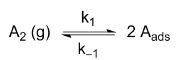If θ denotes the surface coverage and P denotes the pressure, the slope of the plot of 1/θ versus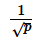is

Solution:
*Answer can only contain numeric values
QUESTION: 39

For a gas phase unimolecular reaction at temperature 298 K, with a pre-exponential factor of 2.17 × 1013 s–1, the entropy of activation (J K–1 mol–1) is _______________

Solution:
*Answer can only contain numeric values
QUESTION: 40

A liquid has vapor pressure of 2.02 ×103 N m–2 at 293 K and heat of vaporization of 41 kJ mol–1. The boiling point of the liquid (in Kelvin) is _______________

Solution:
QUESTION: 41

The rotational partition function of a diatomic molecule with energy levels corresponding to J = 0 and 1, is (wher e, is a constant)

Solution:
*Answer can only contain numeric values
QUESTION: 42

The internal energy of an i deal gas follows the equation U = 3.5 PV + k, where k is a constant. The gas expands fro m an initial volume of 0.25 m3 to a final volume of 0.86 m3. If the initial pressure is 5 N m–2, the change in internal energy (in Joules) is (given PV1.3 = constant) _______________

Solution:
*Answer can only contain numeric values
QUESTION: 43

Th e solubility product of AgBr(s) is 5×10–13 at 298 K. If the standard reduction potential of the half-cell,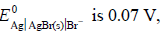, the standard reduction potential,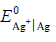(in volts) is _________.

Solution:
*Answer can only contain numeric values
QUESTION: 44

One mole of a substance is heated from 300 K to 400 K at constant pressure. The  CP of the sub stance is given by cp(J-1mol-1)= 5+0.1 T. the change in entropy, in JK-1mol-1, of the substance is ______________

Solution:
QUESTION: 45

h e potential energy (PE) versus reaction coordinate diagrams for electron transfer reactions with rate constants k1, k2 and k3, are given below. The increasing order of the rate constants is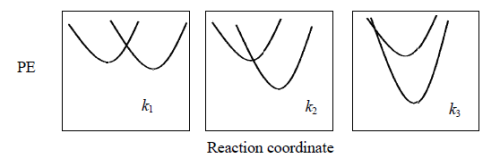Solution:
QUESTION: 46

Th e distance b etween two successive (110) planes in a simple cubic lattice with lattice parameter

‘a’ is

Solution:
QUESTION: 47

The percent transmittance of 8 × 10-5 M solution of KMnO4 is 39.8 when measured at 510 nm in a cell of path length of 1 cm. The absorbance and the molar extinction coefficient (in M-1cm-1) of this solution are, respectively,

Solution:
QUESTION: 48

The value of ‘g’ and the number of signals observed for the reference standard, diphenylpicrylhydrazyl (DPPH), in the solid state ESR spectrum are, respectively

Solution:
QUESTION: 49

Ammonolysis of S2Cl2 in an inert solvent gives

Solution:
QUESTION: 50

The complexes K2[NiF6] and K3[CoF6] are

Solution:
QUESTION: 51

The point group of IF7 is

Solution:
QUESTION: 52

When one CO group is replaced by PPh3 in [Cr(CO)6], which one of the following statements is

TRUE?

Solution:
QUESTION: 53

Identify X in the reaction, [Pt(NH3)4]2+ + 2 HCl X

Solution:
QUESTION: 54

Identify the function of hemocyanin and the metal responsible for it.

Solution:
*Answer can only contain numeric values
QUESTION: 55

The limiting current (in μA) from the reduction of 3 × 10-4 M Pb2+, using a dropping mercury electrode (DME) with characteristics, m = 3.0 mg s-1 and t = 3s, is (diffusion coefficient of Pb2+ = 1.2 × 10-5 cm2 s-1) _______________

Solution:
*Answer can only contain numeric values
QUESTION: 56

The number of possible stereoisomers obtained in the following reaction is _______________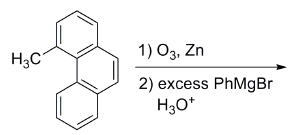Important : you should answer only the numeric value

Solution:
QUESTION: 57

The major product formed in the following reaction is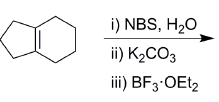Solution:
QUESTION: 58

The most suitable reagent(s) to effect the following transformation is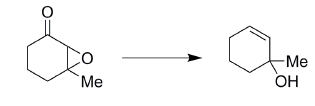Solution:
QUESTION: 59

The major product formed in the following reaction is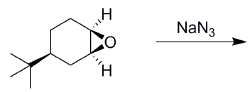Solution:
QUESTION: 60

Solvolysis of the optically active compound X gives, mainly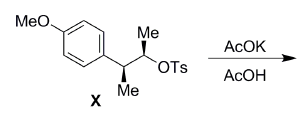Solution:
QUESTION: 61

The major product formed in the following reaction is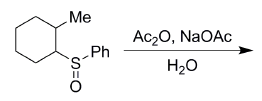Solution:
QUESTION: 62

The tetrapeptide, Ala-Val-Phe-Met, on reaction with Sanger’s reagent, followed by hydrolysis gives

Solution:
QUESTION: 63

The major product formed in the following reaction is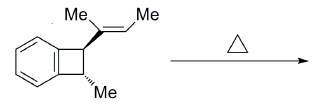Solution:
QUESTION: 64

The Beckmann rearrangement of a bromoacetophenone oxime (C8H8BrNO) gives a major product having the following 1H NMR (δ, ppm): 9.89 (s, 1H), 7.88 (s, 1H), 7.45 (d, 1H, J = 7.2 Hz), 7.17 (m, 1H), 7.12 (d, 1H, J = 7.0 Hz), 2.06 (s, 3H). The structure of the product is

Solution:
QUESTION: 65

The major products, K and L formed in the following reactions are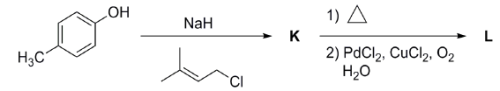Solution: